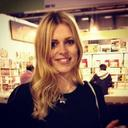# clcclose in the form [x_width y_width …]. regionprops

clcclose allclear all%% Input     I,path=uigetfile({‘*.jpg;*.tif;*.png;*.gif’,’All Image Files’;},’Select an MRI input image’);  %filename = uigetfile displays a modal dialog box that lists files in the current folder and enables you to select or enter the name of a file. If the file name is valid (and the file exists), uigetfile returns the file name as a string when you click Open. If you click Cancel (or the window’s close box), uigetfile returns 0.input_image=strcat(path,I);show_input_image=imread(input_image);figure;imshow(show_input_image);  title(‘Input MRI(Magnetic resonance imaging)image’,’FontSize’,10);%% Filterinput_image_for_filtering=imresize(show_input_image,256,256);if size(input_image_for_filtering,3)>1    input_image_for_filtering=rgb2gray(input_image_for_filtering); % convert to doubleend % convert to double formatI = im2double(input_image_for_filtering);% create maskmask = ones(3,3)/9;% filter imagefinal_filtered_image = filter2(mask,I); figure;imshow(final_filtered_image);title(‘Filtered image After Mean Filter’,’FontSize’,10);imhist(final_filtered_image);%% thresholdingThreshhold_image=imresize(input_image_for_filtering,256,256);t0=60;th=t0+((max(input_image_for_filtering(:))+min(input_image_for_filtering(:)))./2);for a=1:1:size(input_image_for_filtering,1)    for b=1:1:size(input_image_for_filtering,2)        if input_image_for_filtering(a,b)>th            Threshhold_image(a,b)=1;        else            Threshhold_image(a,b)=0;        end    endendfigure;imshow(Threshhold_image);title(‘Threshhold image obtained after thresholding’,’FontSize’,10);imhist(Threshhold_image);%% Morphological Operationlabeled_connected_components_in_2D_binary_image=bwlabel(Threshhold_image); % returns the label matrix L that contains labels for the 8-connected objects found in BW. The label matrix, L, is the same size as BW.Measure_properties_of_image_regions=regionprops(logical(Threshhold_image),’Solidity’,’Area’,’BoundingBox’);% ‘Area’=Returns a scalar that specifies the actual number of pixels in the region.% ‘BoundingBox’ Returns the smallest rectangle containing the region, specified as a 1-by-Q*2 vector, where Q is the number of image dimensions, for example, ul_corner width. ul_corner specifies the upper-left corner of the bounding box in the form x y z …. width specifies the width of the bounding box along each dimension in the form x_width y_width …. regionprops uses ndims to get the dimensions of label matrix or binary image, ndims(L), and numel to get the dimensions of connected components, numel(CC.ImageSize).% ‘Solidity’ Returns a scalar specifying the proportion of the pixels in the convex hull that are also in the region. Computed as Area/ConvexArea.Density=Measure_properties_of_image_regions.Solidity;required_area=Measure_properties_of_image_regions.Area;high_dense_area_in_Brain_MRI_image=Density>0.6;maximum_area=max(required_area(high_dense_area_in_Brain_MRI_image));brain_tumor_label=find(required_area==maximum_area);% returns a vector containing the linear indices of each nonzero element in array X.brain_tumor_detected=ismember(labeled_connected_components_in_2D_binary_image,brain_tumor_label);%  ismember(A,B) returns an array containing logical 1 (true) where the data in A is found in B. Elsewhere, the array contains logical 0 (false).% If A and B are tables or timetables, then ismember returns a logical value for each row. For timetables, ismember takes row times into account to determine equality. The output, Lia, is a column vector.if maximum_area>150   figure;   imshow(brain_tumor_detected)   title(‘tumor alone’,’FontSize’,10);    h = msgbox(‘Benign (non-cancerous)Tumor Detected!!’,’Final Output’); else    h = msgbox(‘No Tumor!!’,’Final Output’);    %disp(‘no tumor’);    return;endimhist(brain_tumor_detected);      %% Bounding boxdesired_box_that_we_want_on_image = Measure_properties_of_image_regions(brain_tumor_label);wanted_Box = desired_box_that_we_want_on_image.BoundingBox;figureimshow(input_image_for_filtering);title(‘Bounding Box’,’FontSize’,10);hold on;rectangle(‘Position’,wanted_Box,’EdgeColor’,’y’);hold off;%% Getting Tumor Outline – image filling, eroding, subtracting% erosion the walls by a few pixelsdilation_Amount_applied_on_image = 5;rounds_each_element_to_nearest_integer = floor(dilation_Amount_applied_on_image); % Y = floor(X) rounds each element of X to the nearest integer less than or equal to that element.l,m = size(brain_tumor_detected);filled_image_after_applying_holes = imfill(brain_tumor_detected, ‘holes’);for a=1:l   for b=1:m       p1=a-rounds_each_element_to_nearest_integer;       p2=a+rounds_each_element_to_nearest_integer;       q1=b-rounds_each_element_to_nearest_integer;       q2=b+rounds_each_element_to_nearest_integer;       if p1<1           p1=1;       end       if p2>l           p2=l;       end       if q1<1           q1=1;       end       if q2>m           q2=m;       end       final_eroded_Image(a,b) = min(min(filled_image_after_applying_holes(p1:p2,q1:q2)));   endendfigureimshow(final_eroded_Image);title(‘Eroded image’,’FontSize’,10);%% subtracting eroded image from original BW imagetumor_outline=brain_tumor_detected;tumor_outline(final_eroded_Image)=0;figure;  imshow(tumor_outline);title(‘Tumor Outline’,’FontSize’,10);%% Display Togetherfiguresubplot(231);imshow(show_input_image);title(‘Input image’,’FontSize’,15);subplot(232);imshow(input_image_for_filtering);title(‘Filtered image’,’FontSize’,15);subplot(233);imshow(Threshhold_image);title(‘Threshold image’,’FontSize’,15);subplot(234);imshow(input_image_for_filtering);title(‘Bounding Box’,’FontSize’,15);hold on;rectangle(‘Position’,wanted_Box,’EdgeColor’,’y’);hold off;subplot(235);imshow(brain_tumor_detected);title(‘Tumor alone’,’FontSize’,15);subplot(236);imshow(tumor_outline);title(‘Tumor Outline’,’FontSize’,15);

xHi!
I'm William!

Would you like to get a custom essay? How about receiving a customized one?

Check it out